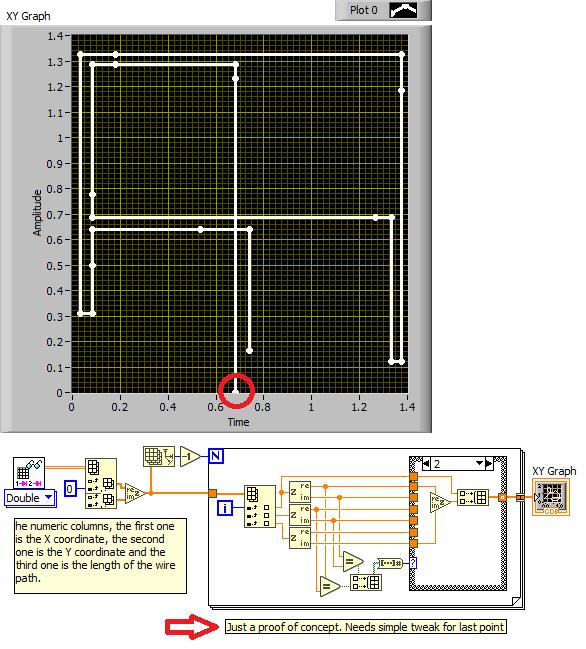# LabVIEW

cancel
Showing results for
Did you mean:

## Generate coordinates of intersection point of two lines

Hi guys, this is my first post 🙂

So basically i have 22 coordinates which i plot into a XY graph to make a "path". The problem is that i am not getting the "path" that i am looking for because i do not have enough coordinates to plot what i want, so i have to create or estimate more coordinates (with the coordinates that i already have) for my graph to be ok.

So i would like to generate the intersection point coordinates of some points so i can have the graph that i want. The first image is the path that i have and the second image is the path that i desire. you can take a look at my VI here.

If it wasnt clear, i would like to know how i can get "automatically" the intersection coordinate of 2 other consecutive coordinates? I started with a case structure to modify the estimation of coordinates, so if i have a D in the text file it means i will have a straight line so i dont need to calculate the intersection point, however if i have a C it means that i want to calculate the intersection point of 2 coordinates.

You can use the text file to get the coordinates i am ploting.

Luis.

Message 1 of 9
(3,949 Views)

## Re: Generate coordinates of intersection point of two lines

• Your file will not read correctly in most places of the world because it has comma as decimal delimiter. Most people cannot use your code unchanged..
• Index array is resizeable.
• Your sequence structure has no purpose.
• You seem to have way too much code.
• Your FOR loop iterates once too many.
• You can use a shift register to get adjacent elements
• You have all right angles, so calculating the intersection is trivial.

I'll have a look at it later, but what point density do you want on your lines? Is it sufficient to define the correct edges?

What's the exact definition of the three numeric columns?LabVIEW Champion.Message 2 of 9
(3,911 Views)

## Re: Generate coordinates of intersection point of two lines

I changed the text file so everyone can use it, and i made the changes you propose.

So to begin with, the numeric columns, the first one is the X coordinate, the second one is the Y coordinate and the third one is the

length of the wire path (physical wire) so the code after unbundel by name is to set the "metric step" between the points i want to plot, then the case structure sets the increment of the points or the path they have to follow  so they can reach each coordinate. So as you can see in the images i posted before, i can plot all right angles but it is manually, like indexing manually every point of each corner, in reallity if you use mi VI you will get the path i have right now, so basically my goal is to get all right angles but automatically.

This Vi i use to get all right angles , but as you can see its ..... well....not that good, but the idea is there.

You can find the coordinates with a point instead of a coma.

Thanks

Luis.

Message 3 of 9
(3,890 Views)

## Re: Generate coordinates of intersection point of two lines

Hi Beer,

I tried to simplify your VI a little bit:Still lot to improve, just wanted to show how to do calculation with (numeric) clusters and where to place constant operations out of loops…

Now it's your turn to add some comments on what to do in each case (C or D) and what is the expected result for each of the cases!

Message 4 of 9
(3,889 Views)

## Re: Generate coordinates of intersection point of two lines

If all turns are +/- 90 degrees, we seems to have an over-determined problem , because the length is already known.LabVIEW Champion.Message 5 of 9
(3,887 Views)

## Re: Generate coordinates of intersection point of two lines

See if this can give you some ideas.... (Yes, you still need to correct for the last point after deciding how if should behave)LabVIEW Champion.Message 6 of 9
(3,860 Views)

## Re: Generate coordinates of intersection point of two lines

Thank you Altenbach,

That gave me some ideas, is there a way to do it not using complex numbers representation?

I am trying to do that but i am not getting any succes in that . What i am getting is a straight line

and not the path i want, i think it should be easy, but i am having troubles to see what i am doing wrong haha.Message 7 of 9
(3,481 Views)

## Re: Generate coordinates of intersection point of two lines

There is nothing special about complex numbers, in fact you could just do a literal translation in a minute starting with my code. Your code is very different, for example it has a FOR loop inside the case structure. Mine doesn't.LabVIEW Champion.Message 8 of 9
(3,384 Views)

## Re: Generate coordinates of intersection point of two lines

Here's the same code without use of complex numbers. Same difference.LabVIEW Champion.Message 9 of 9
(3,376 Views)